Math Practice: Negative and Fractional Exponents

Exponents can be tricky, but even more so when they are negative or fractional. This article begins by reviewing the basic laws of exponents (powers). Then we’ll tackle plenty of practice problems involving negative exponents and fractional exponents.Gravity, the force that holds our solar system together, can be expressed using negative exponents. Fractional exponents play a role in computing the orbital period of a planet.

Image by Comfreak

How Do Exponents Work?

In their simplest form, exponents stand for repeated multiplication. The smallish number (the exponent, or power) located to the upper right of main number (the base) tells how many times to use the base as a factor.

• 32 = 3 × 3 = 9
• 25 = 2 × 2 × 2 × 2 × 2 = 32
• It also works for variables: x3 = (x)(x)(x)

You can even have a power of 1. That just means a single factor of the base: x1 = x.

But what sense can we make out of expressions like 4-3, 253/2, or y-1/6? What does it mean to take “-3” factors of a number? Or “3/2” or “-1/6” of a factor?

It all begins with the Laws of Exponents (Check out: Quick Tips on Using the Exponent Rules.)

The Laws of Exponents

1. Multiplication Law (same bases): xaxb = xa+b
2. Division Law (same bases): xa/xb = xa-b
3. Power Law: (xa)b = xab
4. Multiplication Law (same powers): xaya = (xy)a
5. Division Law (same powers): xa/ya = (x/y)a

Now if we’re going to try to make sense of negative and fractional exponents, then we must at least make sure that our definitions will stay consistent with these Laws of Exponents.

For example, you may already know that the zero exponent on any base results in the number 1 (with one exception: 00 is undefined).

Let’s see if the rule x0 = 1 is consistent with the Laws of Exponents.

• If x0 = 1, then x0xb = 1xb = xb. That’s consistent with the Multiplication Law: x0xb = x0+b = xb
• By the Division Law, if the two exponents happen to be the same, then xa/xa = xa-a = x0 = 1. This certainly agrees with the algebraic fact that dividing a quantity by itself yields 1.

In fact, all of the Laws are consistent with the rule x0 = 1.

Negative Exponents

Consider the Division Law with a = 0.

x0/xb = x0-b = x-b

But remember: x0 = 1. So, reading the above equation backwards, we have discovered the rule for negative exponents!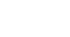Rule for negative exponents

Negative exponents translate to fractions.

For example, 4-3 = 1/(43) = 1/64.

The more negative the exponent, the smaller the value. This is especially important in the sciences when talking about orders of magnitude (how big or small things are). In fact, the positive and negative powers of 10 are essential in scientific notation.

• (giga-) 109 = 1,000,000,000 = 1 billion
• (mega-) 106 = 1,000,000 = 1 million
• (kilo-) 103 = 1000 = 1 thousand
• (Unit – no prefix) 100 = 1
• (milli-) 10-3 = 0.001 = 1 thousandth
• (micro-) 10-6 = 0.000 001 = 1 millionth
• (nano-) 10-9 = 0.000 000 001 = 1 billionth

Fractional Exponents

How can we define fractional exponents so that the Laws of Exponents remain consistent?

Consider any fraction, say 1/2. If you multiply by the denominator, you end up back at the value 1.

(1/2)(2) = 1

Now consider 1/2 and 2 as exponents on a base. For example, with base = 9, we could write:

9(1/2)(2) = 91

The right side is simply equal to 9. But the left side can be rewritten using the Power Law.

(91/2)2 = 9

So, whatever 91/2 is, its square must equal 9. In other words, 91/2 is the square root of 9, that is, 91/2 = 3.

In general, x1/2 is the square root of x.

What’s more, is that it works the same way with fractional exponents of the form 1/n for any number n.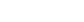Rule for fractional exponents

Fractional exponents translate to roots.

So far, we have rules for exponents like 1/2, 1/3, 1/10, etc. But what about 2/3, 9/4, -11/14, etc.? Again, our Laws of Exponents come to the rescue!

For instance, if you need to know the value of 82/3, then first write 2/3 as a product.

82/3 = 8(1/3)(2) = (81/3)2

Then, work out 81/3, which is by definition the cube root of 8. Since we know that 23 = 8, we have 81/3 = 2.

82/3 = (81/3)2 = (2)2 = 4

In general, you can always express a fractional exponent in terms of roots and powers.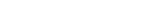General rule for fractional exponents

You may even have to deal with negative fractional exponents. Just think of what each property tells you: Negative exponents translate to fractions, and fractional exponents translate to roots (and powers).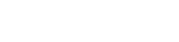General rule for negative fractional exponents

Practice Problems

Now are you ready for some practice?By Agenturfotografin

For each problem below, simplify as much as possible. If the expression has a variable, be sure that your final result has only positive whole exponents. Answers are given at the end.

1. 1254/3
2. 6-2
3. 16-1/4
4. a-1
5. (4x)0
6. 4x0
7. (x2)-8/3
8. (z2 + 25)1/2
9. (1000p6)-1/3
10. (x1/2 + y1/2)2

Solutions

1. 625.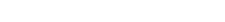2.

3. 1/36. 6-2 = 1/62 = 1/36.
4.

5. 1/2.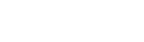6.

7. 1/a. (The power -1 produces the reciprocal of the base.)
8.

9. 1. Anything to the zero power gives the value 1 (as long as the base is nonzero, which we tend to assume in problems like this).
10.

11. 4. Be careful of your order of operations. The zero exponent only applies to x, not the factor of 4 in front of it.
12.

13. Use the Laws of Exponents to simplify.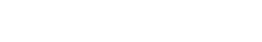14.

15.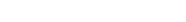.
Yes, this is the final answer! Be careful when working with powers and radicals. You can never break apart a power or radical over a plus or minus! So, the answer is NOT equivalent to z + 5.
16.

17. Laws of Exponents to the rescue again!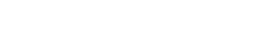18.

19. Just as in Problem 8, you can’t just break up the expression into two terms. In other words, resist the urge to write down x + y as your final answer. I guarantee that you’ll see a big red mark through that result if you do it on the next test.

Instead, think algebraically. Square the binomial first and then rewrite your exponents. (Remember, (a + b)2 = a2 + 2ab + b2.)Author

•Shaun earned his Ph. D. in mathematics from The Ohio State University in 2008 (Go Bucks!!). He received his BA in Mathematics with a minor in computer science from Oberlin College in 2002. In addition, Shaun earned a B. Mus. from the Oberlin Conservatory in the same year, with a major in music composition. Shaun still loves music -- almost as much as math! -- and he (thinks he) can play piano, guitar, and bass. Shaun has taught and tutored students in mathematics for about a decade, and hopes his experience can help you to succeed!